# Three Phase Squirrel Cage Induction Machine

Description of the Three Phase Squirrel Cage Induction Machine component in Schematic Editor.

Table 1. Three Phase Squirrel Cage Induction Machine component in Typhoon HIL Schematic Editor
component component dialog window component parameters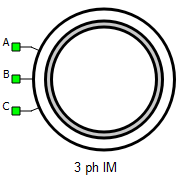• Property tabs:

A, B, and C are the stator winding terminals. The stator winding uses the current source interface. Any change related to the signal processing inputs of the component is documented on the component description window in real time.

## Electrical sub-system model

The electrical part of the machine is represented by the following system of equations, modeled in the stationary αβ reference frame. All rotor variables and parameters are referred to the stator.

$\left[\begin{array}{c}{v}_{\alpha s}\\ {v}_{\beta s}\\ \begin{array}{c}{v}_{\alpha r}^{}\\ {v}_{\beta r}^{}\end{array}\end{array}\right]=\left[\begin{array}{cccc}{R}_{s}& 0& 0& 0\\ 0& {R}_{s}& 0& 0\\ 0& 0& {R}_{r}^{}& 0\\ 0& 0& 0& {R}_{r}^{}\end{array}\right]\left[\begin{array}{c}{i}_{\alpha s}\\ {i}_{\beta s}\\ \begin{array}{c}{i}_{\alpha r}^{}\\ {i}_{\beta r}^{}\end{array}\end{array}\right]+\frac{d}{dt}\left[\begin{array}{c}{\psi }_{\alpha s}\\ {\psi }_{\beta s}\\ \begin{array}{c}{\psi }_{\alpha r}^{}\\ {\psi }_{\beta r}^{}\end{array}\end{array}\right]+\left[\begin{array}{c}0\\ 0\\ \begin{array}{c}{}_{}{\omega }_{r}{\psi }_{\beta r}{}_{}\\ {}_{}{-\omega }_{r}{\psi }_{\alpha r}{}_{}\end{array}\end{array}\right]$

$\left[\begin{array}{c}{\psi }_{\alpha s}\\ {\psi }_{\beta s}\\ \begin{array}{c}{\psi }_{\alpha r}^{}\\ {\psi }_{\beta r}^{}\end{array}\end{array}\right]=\left[\begin{array}{cccc}{L}_{s}& 0& {L}_{m}& 0\\ 0& {L}_{s}& 0& {L}_{m}\\ {L}_{m}& 0& {L}_{r}& 0\\ 0& {L}_{m}& 0& {L}_{r}\end{array}\right]\left[\begin{array}{c}{i}_{\alpha s}\\ {i}_{\beta s}\\ \begin{array}{c}{i}_{\alpha r}^{}\\ {i}_{\beta r}^{}\end{array}\end{array}\right]$

${T}_{e}=\frac{3}{2}p\left({\psi }_{\alpha s}{i}_{\beta s}-{\psi }_{\beta s}{i}_{\alpha s}\right)$

If the saturation effects are considered, the equations have the same form, but in that case the magnetizing flux is a function of the magnetizing current:

${\psi }_{m}=f\left({i}_{m}\right)$

where:

${\psi }_{m}=\sqrt{{\left({\psi }_{\alpha s}+{\psi }_{\alpha r}\right)}^{2}+{\left({\psi }_{\beta s}+{\psi }_{\beta r}\right)}^{2}}$

${i}_{m}=\sqrt{{\left({i}_{\alpha s}+{i}_{\alpha r}\right)}^{2}+{\left({i}_{\beta s}+{i}_{\beta r}\right)}^{2}}$

Table 2. Three Phase Squirrel Cage Induction Machine electrical subsystem model variables
symbol description
ψαs Alpha axis component of the stator flux [Wb]
ψβs Beta axis component of the stator flux [Wb]
ψαr Alpha axis component of the rotor flux, referred to the stator [Wb]
ψβr Beta axis component of the rotor flux, referred to the stator [Wb]
iαs Alpha axis component of the stator current [A]
iβs Beta axis component of the stator current [A]
iαr Alpha axis component of the rotor current, referred to the stator [A]
iβr Beta axis component of the rotor current, referred to the stator [A]
vαs Alpha axis component of the stator voltage [V]
vβs Beta axis component of the stator voltage [V]
vαr Alpha axis component of the rotor voltage, referred to the stator [V]
vβr Beta axis component of the rotor voltage, referred to the stator [V]
Rs Stator phase resistance [Ω]
Rr Rotor phase resistance, referred to the stator [Ω]
Lm Magnetizing (mutual, main) inductance [H]
Ls Stator phase inductance [H] ( $={L}_{ls}+{L}_{m}$ )
Lr Rotor phase inductance, referred to the stator [H] ( $={L}_{lr}+{L}_{m}$ )
ωr Rotor electrical speed [rad/s] ( $=p{\omega }_{m}$ )
p Machine number of pole pairs
Te Machine developed electromagnetic torque [Nm]
ψm Magnetizing flux [Wb]
im Magnetizing current [A]

## Mechanical sub-system model

Motion equation:

Table 3. Mechanical sub-system model variables
symbol description
Jm Combined rotor and load moment of inertia [kgm2]
Te Machine developed electromagnetic torque [Nm]
Tl Shaft mechanical load torque [Nm]
b Machine viscous friction coefficient [Nms]
Note: Motion equation is the same for all of the rotating machine models.

## Electrical

This component offers two levels of model fidelity, designated by the Model Type property. The following options are available:
• linear
• nonlinear
If the selected Model Type is nonlinear, the following saturation types can be specified:
• flux vs current
• absolute inductance vs current
Table 4. Electrical parameters
symbol description
Rs Stator phase resistance [Ω]
Rr Rotor phase resistance, referred to the stator [Ω]
Lls Stator leakage inductance [H]
Llr Rotor leakage inductance, referred to the stator [H]
Lm Magnetizing (mutual, main) inductance [H]
im vector List of instantaneous values of the magnetizing current [A]
psim vector List of instantaneous values of the magnetizing flux [Wb]
Lm vector List of instantaneous values of the magnetizing inductance [H]
The Dynamic resistances checkbox enables real-time change to stator and rotor resistances. If calculations of these resistances depend on the temperature, they can be routed to a load input with signal processing connections, provided that this property is enabled. The vectorized array [Rs, Rr] is in ohms.
Note: This property is only available on linear machines, and fault properties cannot be simulated simultaneously with this property. Additionally, simulation accuracy may deteriorate on smaller simulation time steps for virtual HIL.
The three phase squirrel cage induction machine component can include magnetic saturation effects. In that case, magnetizing flux or magnetizing inductance is defined as a function of the magnetizing current. That function is represented in the form of lookup table. The lookup tables use linear interpolation and linear extrapolation.
Saturation can be parametrized in the following ways:
1. magnetizing flux vs magnetizing current
2. magnetizing inductance vs magnetizing current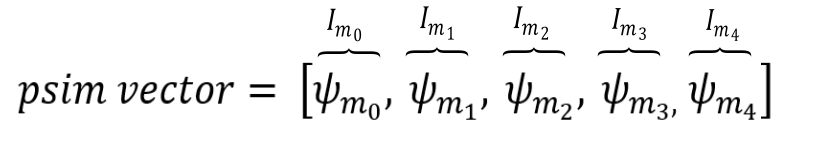Figure 1. Nonlinear Model Type: magnetizing flux as function of magnetizing current, ψm=f(im)
im_vector = [0.0, 0.661428, 0.957988, 1.224002, 1.527775, 1.836498, 2.485056, 3.197537, 4.162313, 5.57879, 8.211348, 12.342407, 22.172606]
psim_vector = [0.0, 0.125279, 0.192308, 0.25488, 0.318532, 0.382499, 0.511695, 0.635623, 0.76725, 0.885866, 1.007544, 1.097936, 1.186302]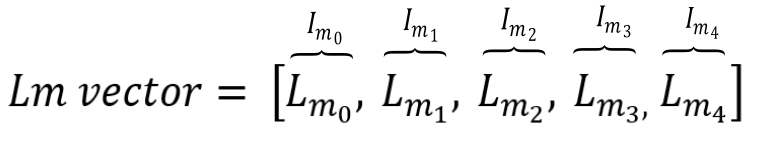Figure 2. Nonlinear Model Type: magnetizing inductance as function of magnetizing current, Lm=f(im)
im_vector = [0.0, 0.661428, 0.957988, 1.224002, 1.527775, 1.836498, 2.485056, 3.197537, 4.162313, 5.57879, 8.211348, 12.342407, 22.172606]
Lm_vector = [0.0, 0.189407, 0.200741, 0.208235, 0.208494, 0.208277, 0.205909, 0.198785, 0.184332, 0.158792, 0.122701, 0.088956, 0.053503]

## Mechanical

Table 5. Mechanical parameters
symbol description
pms Machine number of pole pairs
Jm Combined rotor and load moment of inertia [kgm2]
Friction coefficient Machine viscous friction coefficient [Nms]
Unconstrained mechanical angle Limiting mechanical angle between 0 and 2π

symbol description
Load source Load can be set from SCADA/external or from model (in model case, one signal processing input will appear)
Load ai offset Assigned offset value to the input signal representing external torque command
Load ai gain Assigned gain value to the input signal representing external torque command

External load enables you to use an analog input signal from a HIL analog channel with the load_ai_pin address as an external torque/speed load, and to assign offset (V) and gain (Nm/V) to the input signal, according to the formula:

${T}_{l}=load_ai_gain·\left(AI\left(load_ai_pin\right)+load_ai_offset\right)$

Note: An analog input pin may be overwritten if another component uses the same analog input pin. If another property (from the same or a different component) uses the same analog input pin, the input signal value will be applied to only one of those properties. For instance, if both the load and the resolver carrier signal use the same analog input pin, the signal value will only be applied only to one of these.

## Feedback

Table 7. Feedback parameters
symbol description
Encoder ppr Incremental encoder number of pulses per revolution
Resolver pole pairs Resolver number of pole pairs
Resolver carrier source Resolver carrier signal source selection (internal or external)
External resolver carrier source type External resolver carrier signal source type selection (single ended or differential); available only if the Resolver carrier source property is set to external
Resolver carrier frequency Resolver carrier signal frequency (internal carrier) [Hz]
Resolver ai pin 1 Resolver carrier input channel 1 address (external carrier)
Resolver ai pin 2 Resolver carrier input channel 2 address (external carrier); available only if the External resolver carrier source type property is set to differential
Resolver ai offset Resolver carrier input channel offset (external carrier)
Resolver ai gain Resolver carrier input channel gain (external carrier)
Absolute encoder protocol Standardized protocol providing the absolute machine encoder position

If an external resolver carrier source is selected, the source signal type can be set as either single ended or differential. The single ended external resolver carrier source type enables use of an analog input signal from the HIL analog channel with the res_ai_pin_1 address as the external carrier source. Additionally, offset (V) and gain (V/V) values can be assigned to the input signal, according to the formula:

$res_carr_src=res_ai_gain·\left(AI\left(res_ai_pin_1\right)+res_ai_offset\right)$

The differential external resolver carrier source type enables use of two analog input signals from the HIL analog channels with the res_ai_pin_1 and the res_ai_pin_2 addresses. Analog signals from these HIL analog inputs are subtracted, and the resulting signal is used as the external differential carrier source. Additionally, offset (V) and gain (V/V) values can be assigned to the input signal (similarly to the single ended case), according to the formula:

$res_carr_src=res_ai_gain·\left(\left(AI\left(res_ai_pin_1\right)-AI\left(res_ai_pin_2\right)\right)+res_ai_offset\right)$
Note: Differential external resolver signal support is available from the 2023.1 software version. Only the single ended external resolver option was available in previous software versions.
Note: In order to get a resolver signal with an amplitude of 1 when using an external carrier signal, the offset and the gain should be chosen in such a way that the resolver carrier signal has an amplitude of 1 after the adjustment. As shown in Figure 1, the sinusoidal signal used to generate external resolver carrier source is fed to HIL analog input 1. The analog input signal is scaled in order to get the resolver signals with an amplitude of 1.
Note: An analog input pin may be overwritten if another component uses the same analog input pin. If another property (from the same or a different component) uses the same analog input pin, the input signal value will be applied to only one of those properties. E.g. if both the load and the resolver carrier signal use the same analog input pin, the signal value will be applied only to one of these.

The following expression must hold in order to properly generate the encoder signals:

$4·enc_ppr·{f}_{m}{·T}_{s}\le 1$
Table 8. Variables in the encoder limitation expression
symbol description
enc_ppr Encoder number of pulses per revolution
fm Rotor mechanical frequency [Hz]
Ts Simulation time step [s]
Note: While the machine speed is positive, the encoder channel B signal leads the encoder channel A signal.
Note: Absolute encoder protocol is not supported on HIL402 (configurations: 1, 2, 3, and 4).

## Snubber

All machines with current source based circuit interfaces have the Snubber tab in the properties window where the value of snubber resistance can be set. Snubbers are necessary in the cases when an inverter or a contactor is directly connected to the machine terminals. This value can be set to infinite (inf), but it is not recommended when a machine is directly connected to the inverter since there will be a current source directly connected to an open switch. In this case, one of each switch pairs S1 and S2, S3 and S4, and S5 and S6 will be forced closed by the circuit solver in order to avoid the topological conflicts. On the other hand, with finite snubber values, there's always a path for the currents Ia and Ib, so all inverter switches can be open in this case. Circuit representations of this circuit without and with snubber resistors are shown in Figure 3 and Figure 4 respectively. Snubbers are connected across the current sources.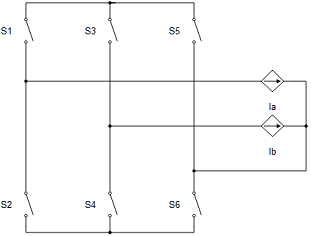Figure 5. Circuit representation of machine and inverter when all the switches are open without resistors (not recommended)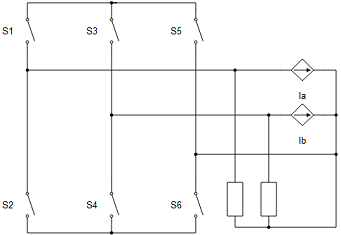Figure 6. Circuit representation of machine and inverter when all the switches are open with resistors (recommended)
Note: Snubbers exist only in the machine components that have the current source based circuit interface.
Note: Snubbers are dynamic, which means that the snubber is dynamically added to circuit modes where topological conflicts are detected.
Table 9. Snubber parameters
symbol description
Rsnb stator Stator snubber resistance value [Ω]

## Output

This block tab enables a single, vectorized signal output from the machine. The output vector contains selected machine mechanical and/or electrical variables in the same order as listed in this tab.

Note: All machine components have the Execution rate, Electrical torque, Mechanical speed, and Mechanical angle, but the rest of the signals differ from component to component.
Table 10. Output parameters
symbol description
Execution rate Signal processing output execution rate [s]
Electrical torque Machine electrical torque [Nm]
Mechanical speed Machine mechanical angular speed [rad/s]
Mechanical angle Machine mechanical angle [rad]
Stator alpha axis current Alpha axis component of the stator current [A]
Stator beta axis current Beta axis component of the stator current [A]
Rotor alpha axis current Alpha axis component of the rotor current, referred to the stator [A]
Rotor beta axis current Beta axis component of the rotor current, referred to the stator [A]
Stator alpha axis flux Alpha axis component of the stator flux [Wb]
Stator beta axis flux Beta axis component of the stator flux [Wb]
Rotor alpha axis flux Alpha axis component of the rotor flux, referred to the stator [Wb]
Rotor beta axis flux Beta axis component of the rotor flux, referred to the stator [Wb]

## Fault

In this block tab, you can choose the fault simulation and specify the type of fault. The chosen fault can be in one of the phases. When choosing the fault, a machine input terminal is either created or extended by a new vectorized input for defining fault parameters. For the ITSC (Interturn Short Circuit) fault type, the vectorized array is [severity factor, Rf]. The machine component fault tab layout is shown here:Figure 7. Three Phase Squirrel Cage Induction Machine fault tab properties
Table 11. Three Phase Squirrel Cage Induction Machine fault parameters
symbol description
Fault Simulation Enabling input for fault parameters
Fault Type Type of the fault to be simulated
Fault Location Phase in which the fault is simulated
Execution rate Signal processing execution rate at which the model matrices are calculated [s]

## Electrical sub-system model for a machine with an ITSC fault in phase a

Table 12. Three Phase Squirrel Cage Induction Machine fault model variables and parameters
symbol description
vαs Alpha axis component of the stator voltage [V]
vβs Beta axis component of the stator voltage [V]
vαs2 Voltage of the short circuited turns in the phase a [V]
iαs Alpha axis component of the stator current [A]
iβs Beta axis component of the stator current [A]
iαr Alpha axis component of the rotor current, referred to the stator [A]
iβr Beta axis component of the rotor current, referred to the stator [A]
if Fault current [A]
ψαs Alpha axis component of the stator flux [Wb]
ψβs Beta axis component of the stator flux [Wb]
ψαr Alpha axis component of the rotor flux, referred to the stator [Wb]
ψβr Beta axis component of the rotor flux, referred to the stator [Wb]
ψαs2 Flux of the short circuited turns in the phase a [Wb]
Rs Stator phase resistance [Ω]
Rr Rotor phase resistance, referred to the stator [Ω]
Rf Fault resistance (minimum value 0, maximum value 100 Ω)
µ Fault severity factor - short-circuited number of turns (minimum value 1e-4, maximum value 1)
Lls Stator leakage inductance [H]
Lm Magnetizing (mutual, main) inductance [H]
Ls Stator phase inductance [H] ( $={L}_{ls}+{L}_{m}$ )
Lr Rotor phase inductance, referred to the stator [H] ( $={L}_{lr}+{L}_{m}$ )
ωr Rotor electrical speed [rad/s] ( $=p{\omega }_{m}$ )